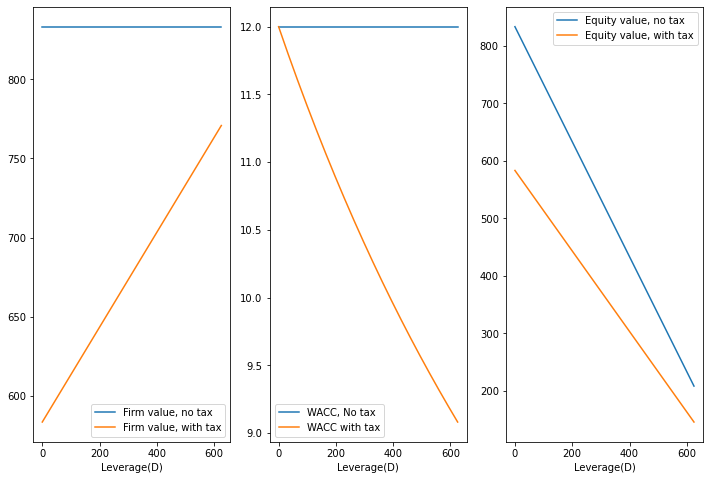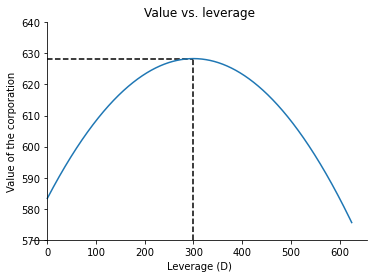# WACC musings¶

Section 4.4 of my notes provides proofs that WACC is the appropriate discount rate for unlevered FCFF in the world of Miller and Modigliani, with and without taxes. This notebook replicates the numerical illustrations of this fact we provided in class.

First we import the modules we will need.

In :
import pandas as pd # pandas is excellent for creating and manipulating dataframes, R-style
import numpy as np # great for array manipulations and simulations
import matplotlib.pyplot as plt #graphing module with matlab-like properties
%matplotlib inline


Now we set parameters and perform a couple of preliminary computations.

In :
Ebitlow=50 # Ebit in bad times
Ebithigh=150 # Ebit in good times
phigh=0.5 # frequency of good times
rD=0.08 # interest rate on debt
ErU=0.12 # unlevered equity return
tau=0.3 # tax rate on EBIT

n=20 # number of grid points for debt levels

# first we compute the value of the unlevered corporation and the max debt level

Ebit=phigh*Ebithigh+(1-phigh)*Ebitlow # expected EBIT each period
Vunotax=Ebit/ErU
Vutax=(1-tau)*Vunotax

Dmax=Ebitlow/rD

# debt grid

Dgrid=np.array([i*Dmax/(n-1) for i in range(n)])


Now we make tables of values with and without taxes.

In :
pd.options.display.float_format = '{:,.2f}'.format # this is the financial format we like

Vnotax=pd.DataFrame({'D':Dgrid}) # no tax
Vtax=pd.DataFrame({'D':Dgrid}) # with tax

Vnotax['V(D)']=Vunotax # value of corporation if no tax
Vtax['V(D)']=np.array([Vutax+Dgrid[i]*tau for i in range(n)]) # value of corporation if  tax

Vnotax['E']=Vnotax['V(D)']-Vnotax['D'] # equity value with no tax
Vtax['E']=Vtax['V(D)']-Vtax['D'] # equity value with tax

Vnotax['E(rE)']=100*(Ebit-Vnotax['D']*rD)/Vnotax['E'] # return on equity if no tax
Vtax['E(rE)']=100*(1-tau)*(Ebit-Vtax['D']*rD)/Vtax['E'] # return on equity with tax

Vnotax['WACC']=(Vnotax['E']*Vnotax['E(rE)']+Vnotax['D']*100*rD)/Vnotax['V(D)'] # wacc if no tax
Vtax['WACC']=(Vtax['E']*Vtax['E(rE)']+Vtax['D']*100*rD*(1-tau))/Vtax['V(D)'] # wacc with tax

# the following is a check on our basic leverage formula
Vnotax['E(rE) check']=100*ErU + Vnotax['D']/Vnotax['E']*100*(ErU-rD)# another way to check E(rE) with no tax
Vtax['E(rE) check']=100*ErU + (1-tau)*Vtax['D']/Vtax['E']*100*(ErU-rD) # another way to check E(rE) with tax

# the following is a check that discounting unlevered FCFF at WACC gives the right answer in both cases
Vnotax['V(D) check']=Ebit/(Vnotax['WACC']/100)# another way to check V(D) without tax
Vtax['V(D) check']=(1-tau)*Ebit/(Vtax['WACC']/100) # another way to check V(D) with  tax

print('Value calculations with no tax')
print(Vnotax)
print('\n')
print('Value calculations with taxes')
print(Vtax)

Value calculations with no tax
D   V(D)      E  E(rE)  WACC  E(rE) check  V(D) check
0    0.00 833.33 833.33  12.00 12.00        12.00      833.33
1   32.89 833.33 800.44  12.16 12.00        12.16      833.33
2   65.79 833.33 767.54  12.34 12.00        12.34      833.33
3   98.68 833.33 734.65  12.54 12.00        12.54      833.33
4  131.58 833.33 701.75  12.75 12.00        12.75      833.33
5  164.47 833.33 668.86  12.98 12.00        12.98      833.33
6  197.37 833.33 635.96  13.24 12.00        13.24      833.33
7  230.26 833.33 603.07  13.53 12.00        13.53      833.33
8  263.16 833.33 570.18  13.85 12.00        13.85      833.33
9  296.05 833.33 537.28  14.20 12.00        14.20      833.33
10 328.95 833.33 504.39  14.61 12.00        14.61      833.33
11 361.84 833.33 471.49  15.07 12.00        15.07      833.33
12 394.74 833.33 438.60  15.60 12.00        15.60      833.33
13 427.63 833.33 405.70  16.22 12.00        16.22      833.33
14 460.53 833.33 372.81  16.94 12.00        16.94      833.33
15 493.42 833.33 339.91  17.81 12.00        17.81      833.33
16 526.32 833.33 307.02  18.86 12.00        18.86      833.33
17 559.21 833.33 274.12  20.16 12.00        20.16      833.33
18 592.11 833.33 241.23  21.82 12.00        21.82      833.33
19 625.00 833.33 208.33  24.00 12.00        24.00      833.33

Value calculations with taxes
D   V(D)      E  E(rE)  WACC  E(rE) check  V(D) check
0    0.00 583.33 583.33  12.00 12.00        12.00      583.33
1   32.89 593.20 560.31  12.16 11.80        12.16      593.20
2   65.79 603.07 537.28  12.34 11.61        12.34      603.07
3   98.68 612.94 514.25  12.54 11.42        12.54      612.94
4  131.58 622.81 491.23  12.75 11.24        12.75      622.81
5  164.47 632.68 468.20  12.98 11.06        12.98      632.68
6  197.37 642.54 445.18  13.24 10.89        13.24      642.54
7  230.26 652.41 422.15  13.53 10.73        13.53      652.41
8  263.16 662.28 399.12  13.85 10.57        13.85      662.28
9  296.05 672.15 376.10  14.20 10.41        14.20      672.15
10 328.95 682.02 353.07  14.61 10.26        14.61      682.02
11 361.84 691.89 330.04  15.07 10.12        15.07      691.89
12 394.74 701.75 307.02  15.60  9.97        15.60      701.75
13 427.63 711.62 283.99  16.22  9.84        16.22      711.62
14 460.53 721.49 260.96  16.94  9.70        16.94      721.49
15 493.42 731.36 237.94  17.81  9.57        17.81      731.36
16 526.32 741.23 214.91  18.86  9.44        18.86      741.23
17 559.21 751.10 191.89  20.16  9.32        20.16      751.10
18 592.11 760.96 168.86  21.82  9.20        21.82      760.96
19 625.00 770.83 145.83  24.00  9.08        24.00      770.83


A couple plots we did in class.

In :
fig, axs  = plt.subplots(1,3,figsize=(12, 8))

axs.plot(Vnotax['D'],Vnotax['V(D)'],label='Firm value, no tax')
axs.plot(Vnotax['D'],Vtax['V(D)'],label='Firm value, with tax')
#axs.set_ylabel('Firm value')
axs.set_xlabel('Leverage(D)')
axs.legend()

axs.plot(Vnotax['D'],Vnotax['WACC'],label='WACC, No tax')
axs.plot(Vnotax['D'],Vtax['WACC'],label='WACC with tax')
#axs.set_ylabel('WACC')
axs.set_xlabel('Leverage(D)')
axs.legend()

axs.plot(Vnotax['D'],Vnotax['E'],label='Equity value, no tax')
axs.plot(Vnotax['D'],Vtax['E'],label='Equity value, with tax')
#axs.set_ylabel('Firm value')
axs.set_xlabel('Leverage(D)')
axs.legend()

Out:
<matplotlib.legend.Legend at 0x2111e328dc0>Now assume that expected bankrupcty costs are $0.0005 * D^2$. What is the optimal capital structure? Let's look at it graphically first.

In :
def objective(d):
return -(Vutax+tau*d -0.0005*d**2)

# let's find the optimal capital structure so we can plot it our upcoming chart

from scipy.optimize import minimize
from scipy.optimize import Bounds

bounds=[(0,Dmax)]

res = minimize(objective, 0, method='trust-constr',
options={'verbose': 1}, bounds=bounds)

#let's plot the problem we just solved

dplot=np.arange(start=0,stop=Dmax,step=0.1)

plt.plot(dplot,-objective(dplot))
ax = plt.gca()
ax.set_ylabel('Value of the corporation')
ax.set_xlabel('Leverage (D)')
plt.title('Value vs. leverage')

plt.vlines(res.x, 570, -res.fun, linestyle="dashed")
plt.hlines(-res.fun, 0, res.x, linestyle="dashed")
plt.ylim(570,640)

ax.spines['right'].set_color('none')
ax.spines['top'].set_color('none')
ax.xaxis.set_ticks_position('bottom')
ax.spines['left'].set_position(('data',0))

gtol termination condition is satisfied.
Number of iterations: 10, function evaluations: 14, CG iterations: 6, optimality: 1.67e-09, constraint violation: 0.00e+00, execution time: 0.19 s.Obvioulsy we are looking for the top of that hill. We could use a fine grid in the tables above but, instead, we just cut through the chase and used Python's optimization routine, so we can just print out the bottom line.

In :
print('The optimal level of debt is %.2f.' %res.x)
print('The value of the corporation as the optimal capital structure is %.2f.' %-res.fun)

The optimal level of debt is 300.00.
The value of the corporation as the optimal capital structure is 628.33.